# Diédrico System: Theorem of the three perpendicular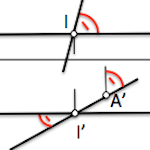One of the most important theorems of descriptive geometry is the so-called “Theorem of the three perpendicular”, It establishes a relation between two lines perpendicular when one of them is parallel to a plane of projection.

This theorem is only applicable for cylindrical orthogonal projections, Although figures of analysis used in their demonstration will be useful later when you define the concept of maximum slope line.

If two lines (to) and (b) they are perpendiculares each other, and one of them (b) It is parallel to a plane of projection,the orthogonal projections of such straight on this plane of projection is perpendicular.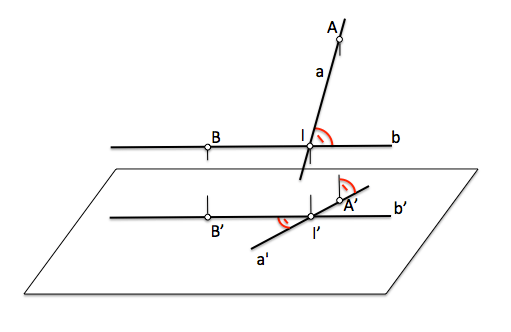To prove this theorem we will have to rely on spatial geometry, in particular will use concepts associated with the perpendicularity between straight and flat that we offered already feel when studying the Fundamentals del System dihedral.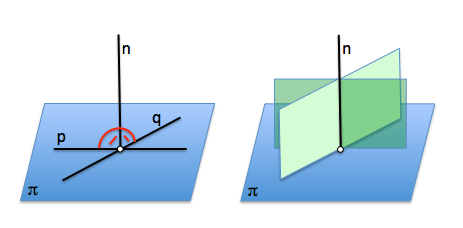A line is perpendicular to a plane if it is with two parallel lines contained in the plane.

If a line is perpendicular to a plane, all planes that contain it are also orthogonal to the plane.

To prove the theorem of the three perpendicular we will assume that we have a plane projected onto another (for example, we will show on a Horizontal H a plane O). The straight intersection “h” It coincides with its projection and we can consider that it is parallel to the plane of projection H.It We project a point “A” on the plane of projection plane. the straight a-a.’ is perpendicular to the plane of projection.Any plane containing to the straight a-a.’ must be perpendicular to the plane Horizontal H projection. If we consider a plane containing this straight, and is perpendicular to the straight line h, is also orthogonal to the plane O (and any plane containing h)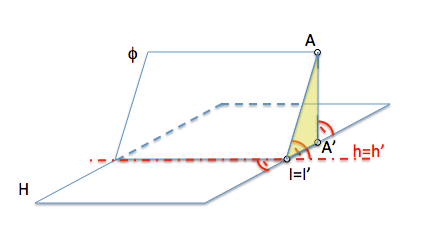The new plane perpendicular to H and a O cuts to these plans in the straight a-ES and A'-I’ It will be therefore orthogonal to the overlapping lines h and h’.

We can see the three orthogonality conditions that give their name to this theorem.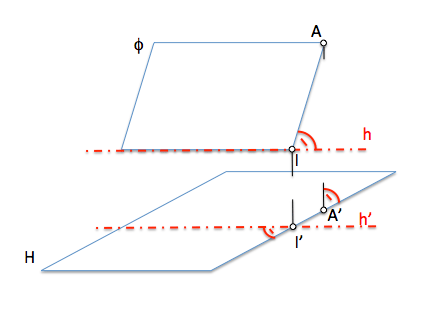If we separate the plane O, moving it according to the direction normal to the plane of projection H, We will see that the straight line h separates from its projection h’ remaining parallel to the plane H. In these circumstances we will see that the straight orthogonal I-A to “h” is projected as I'-TO’ orthogonal to h’, verifying the theorem of three perpendicular.Sistemas_de_representacion